Kotlin: An Illustrated Guide • Chapter 2

# FunctionsIn the last chapter, we wrote some Kotlin code to calculate the circumference of a circle. In this chapter, we’re going to write some functions that will make it easy to calculate the circumference of any circle!

If you’re just landing here and you’re new to the Kotlin language, be sure to head back to chapter one and catch up!

## Functions

As we saw in the last chapter, calculating the circumference of a circle is easy:And here’s some Kotlin code that we wrote to do that calculation:

``````val pi = 3.14
val circumference = 2 * pi * radius``````

That code calculates the circumference of a circle that has a radius of 5.2. But of course, not all circles have a radius of 5.2! What happens if we also want to determine the circumference of a circle that has a radius of 6.7? Or 10.0?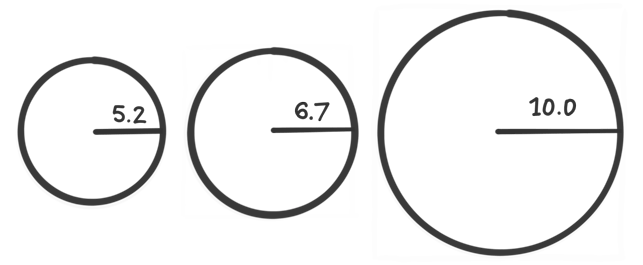Well, we could just write out the equation multiple times.

``````val pi = 3.14

val circumferenceOfSmallCircle = 2 * pi * radius

val circumferenceOfMediumCircle = 2 * pi * radius

val circumferenceOfLargeCircle = 2 * pi * radius``````

This certainly works, but wow - look at how we had to type the same thing over and over again!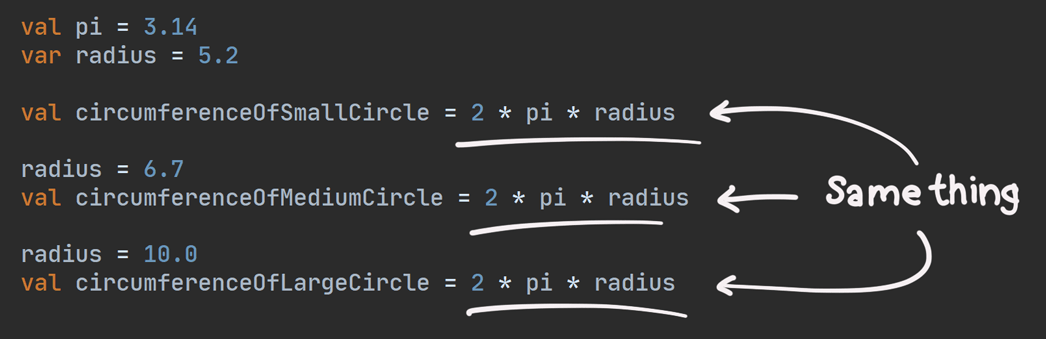When we have the same code over and over again like this, we call it duplication. In most cases, duplicated code is bad because:

1. When you type the same thing so many times, it becomes more likely that you might type it wrong in one of those cases. For example, one time, you might accidentally type `3 * pi radius`.
2. If you want to change the equation, you have to find all of the places where you typed it, and make sure you update each one of them.
3. It can be more difficult to read when your eyes see the same thing written over and over again on the screen.

Let’s change our code so that we only have to write `2 * pi * radius` one time, and then use that to calculate the circumference of any circle. In other words, let’s remove the duplication!

## Removing Duplication with Functions

Even though we wrote `2 * pi * radius` three times, the only value that actually changed each time was `radius`. In other words, `2` never changed, and `pi` never changed (it equaled `3.14` each time). But the value of `radius` was different each time: first `5.2`, then `6.7`, and then `10.0`.

Since the radius is the only thing that changes each time, it would be awesome if we could just convert a radius into a circumference. In other words, what if we could build a machine where we insert a radius on one side, and a circumference pops out of the other side?• Since we’re putting in a radius, we might call that the input.
• And since a circumference comes out of the other side, we might call that the output.

Now, we won’t be creating a real machine, but instead, we will create a function, which will do exactly what we want - we’ll give it a radius and get a circumference back from it!

## Function Basics

### Creating a Function

Here’s how you can write a simple function in Kotlin:This looks like a lot, but there are really just a few pieces, and they’re all easy to understand.

1. `fun` is a keyword (just like `val` and `var` are keywords). It tells Kotlin that you are writing a function.
2. `circumference` is the name of our function. We can name the function just about anything we want, but here, I chose `circumference`.
3. `(radius: Double)` says that this function has an input called `radius`, which has a type called `Double`. We call `radius` a parameter of this function.
4. `: Double` after the closing parenthesis indicates that the output of the function will be of type `Double`. This is called the return type of the function.
5. `2 * pi * radius` is called the body of the function. Whatever this expression evaluates to will be the output of the function. The value of that output is referred to as the result of the function. It’s the thing that comes out of the machine. Note that whatever this expression evaluates to must be the same type that’s specified by the return type. In this example, `2 * pi * radius` must evaluate to a `Double` or we’ll get an error.

Let’s compare our function with the machine we imagined above:You might recall from the last chapter that you often don’t have to specify the type of a variable, and instead let Kotlin use its type inference. You can also use type inference when writing functions like this. Just leave off the return type, so that it looks like this:

``fun circumference(radius: Double) = 2 * pi * radius``

Kotlin programmers will often use type inference for simple functions like this one.

And now that we’ve created our function, it’s time to use it!

### Calling a Function

When you use the function - that is, when you put something into the machine - it’s referred to as calling or invoking the function. The place where it is called is referred to as the calling code or the call site.

Here’s how you call a function in Kotlin: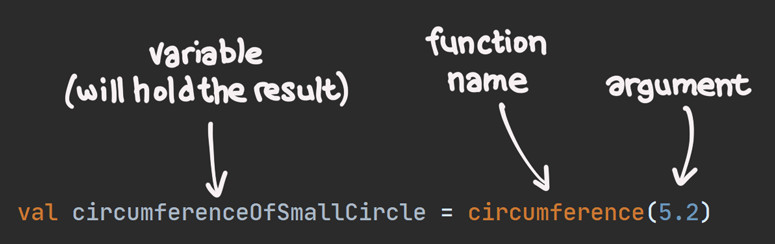1. `circumferenceOfSmallCircle` is a variable that will hold the result of the function call (that is, whatever comes out of the machine).
2. `circumference` is the name of the function that we’re calling.
3. The value `5.2` is the argument of this function - it’s the thing that we’re putting into the machine. When we call a function with an argument, we sometimes say that we are passing the argument to the function.

From this point forward, I’ll usually include the parentheses after the name of a function. For example, I’ll refer to it as `circumference()` rather than just `circumference`. This is done to help make it clear that I’m referring to a function.

What happens when you call a function? Let’s say we wrote a function and called it, like this:

``````fun circumference(radius: Double) = 2 * pi * radius

val circumferenceOfSmallCircle = circumference(5.2)``````

When we call that function, it’s kind of like we’re just plopping the body of the function - `2 * pi * radius` - right where we see the function call - `circumference(5.2)`.

So you can imagine it like this:

``````fun circumference(radius: Double) = 2 * pi * radius

val circumferenceOfSmallCircle = 2 * pi * radius``````

Then, since we passed `5.2` as the radius, we could imagine substituting the value `5.2` where we had plopped in `radius`:

``````fun circumference(radius: Double) = 2 * pi * radius

val circumferenceOfSmallCircle = 2 * pi * 5.2``````

In summary, when we call `circumference(5.2)`, it’s as if we had written `2 * pi * 5.2` at the same spot.

Now that we’ve got a function that can calculate the circumference from a radius, we can call that function as many times as we need!

``````val pi = 3.14

val circumferenceOfSmallCircle = circumference(5.2)
val circumferenceOfMediumCircle = circumference(6.7)
val circumferenceOfLargeCircle = circumference(10.0)``````

Doesn’t this look nicer than Listing 2.2? Because we have a function, we don’t need to write `2 * pi * radius` over and over! Instead, we just call the `circumference()` function once for each circle.

#### Arguments and Parameters: What’s the difference?

It’s easy to confuse an argument with a parameter, so it’s important to understand the distinction:

1. An argument is a value that you pass to the function. For example, you might pass `5.2` into the `circumference()` function. The argument is `5.2`.
2. A parameter is a variable inside the function that will hold that value. For example, when you pass `5.2` to `circumference()`, it is assigned to the parameter called `radius`.

For an easy way to remember the difference, check out this mnemonic.

## Functions with More Than One Parameter

The `circumference()` function has just one parameter, called `radius`, but there are times when you might need a function that has more than that. Let’s create a function that has two parameters!

Even if physics class was a while ago, you probably know how to calculate speed. It’s easy to remember, because we say it aloud all the time - “The speed limit is 100 kilometers per hour”.

“Kilometers per hour” is just distance (“kilometers”) divided by (“per”) time (“hour”).In Kotlin, you use a forward slash to represent division. You can think of it as a fraction that fell over to the left: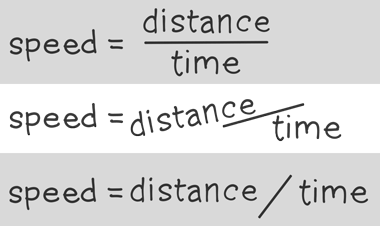To start with, let’s just write some simple code to calculate the average speed of a car that has traveled 321.8 kilometers in 4.15 hours.

``````val distance = 321.8
val time = 4.15
val speed = distance / time``````

Now, let’s turn that `distance / time` expression into a function. In order to create a function for speed, we need to know two things: `distance` and `time`.

In Kotlin, when you need a function with two parameters, you can just separate those parameters with a comma, like this:

``fun speed(distance: Double, time: Double) = distance / time``

When you need to call this function, the arguments are also separated with a comma. For example, we can call the `speed()` function with the same values as we used above, separating those values with a comma:

``val averageSpeed = speed(321.8, 4.15)``

The result is about `77.54` kilometers per hour.

Note that the arguments here are in the same order as the parameters.

• Since `321.8` is the first argument, `321.8` will be assigned to the first parameter, which is `distance`.
• Since `4.15` is the second argument, `4.15` will be assigned to the second parameter, which is `time`.

In other words, the position of the argument matters when we call a function this way, which is why we sometimes refer to arguments like these as positional arguments.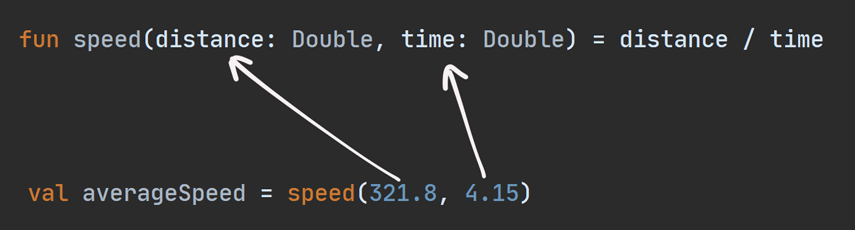But this isn’t the only way to pass arguments to a function!

## Named Arguments

Rather than relying on the position of the arguments, you can instead use the name of the parameter, like this:

``val averageSpeed = speed(distance = 321.8, time = 4.15)``

These are called named arguments. The neat thing about named arguments is that the order doesn’t matter. So, you can call the function like this, with the arguments in a different order:

``val averageSpeed = speed(time = 4.15, distance = 321.8)``

In other words, all five of these function calls will end up with the exact same result:

``````val averageSpeed1 = speed(321.8, 4.15)
val averageSpeed2 = speed(distance = 321.8, 4.15)
val averageSpeed3 = speed(321.8, time = 4.15)
val averageSpeed4 = speed(distance = 321.8, time = 4.15)
val averageSpeed5 = speed(time = 4.15, distance = 321.8)``````

## Default Arguments

In some cases, you might find yourself passing the same argument value to a function over and over again. For example, maybe you’re calculating how fast:

1. A person is walking
2. Another person is biking
3. A third person is driving a car, and
4. A fourth person is flying a plane.Everybody was moving for 2.0 hours, except for the plane, which got to its final destination in only 1.5 hours. Using our `speed()` function from above, you can calculate the speeds like this:

``````val walkingSpeed = speed(10.2, 2.0)
val bikingSpeed = speed(29.6, 2.0)
val drivingSpeed = speed(225.3, 2.0)
val flyingSpeed = speed(1368.747, 1.5)``````

Instead of having to pass `2.0` for the `time` parameter over and over, you could choose to make `2.0` a default argument when we define the function.

Let’s update our `speed()` function so that the `time` parameter defaults to `2.0`.

``fun speed(distance: Double, time: Double = 2.0) = distance / time``

Now, we can omit the argument for `time` whenever it should equal `2.0`, like this:

``````val walkingSpeed = speed(10.2)
val bikingSpeed = speed(29.6)
val drivingSpeed = speed(225.3)
val flyingSpeed = speed(1368.747, 1.5)``````

For walking, biking, and driving, we left off the `time` argument, so those defaulted to `2.0`. But for flying, we passed `1.5`. The results for Listing 2.16 are exactly the same as those for Listing 2.14.

Easy!

But what happens when you want a default argument for the first parameter instead of the second parameter?

### When a Default Argument Comes First

Our walker, biker, driver, and pilot are at it again. But this time, it’s a race! Whoever gets to the finish line 42.195 kilometers away gets the prize.

Everyone finished the race, except for the airplane, which got a flat tire before it could get off the ground:

``````val walkingSpeed = speed(42.195, 8.27)
val bikingSpeed = speed(42.195, 2.85)
val drivingSpeed = speed(42.195, 0.37)
val flyingSpeed = speed(0.12, 0.01)``````

Instead of setting a default `time`, let’s set a default `distance` of `42.195`:

``fun speed(distance: Double = 42.195, time: Double) = distance / time``

Since the walker, the biker, and the driver all travel the same distance, we should be able to omit the value for the first parameter, `distance`. You might be tempted to call the function like this:

``````val walkingSpeed = speed(8.27)
val bikingSpeed = speed(2.85)
val drivingSpeed = speed(0.37)
val flyingSpeed = speed(0.12, 0.01)``````
Error

But this causes an error: No value passed for parameter ‘time’. Why did that happen?

Since we’re using positional arguments, we actually ended up omitting time instead of distance.

In other words, we wanted to assign `8.27` to the `time` parameter, like this: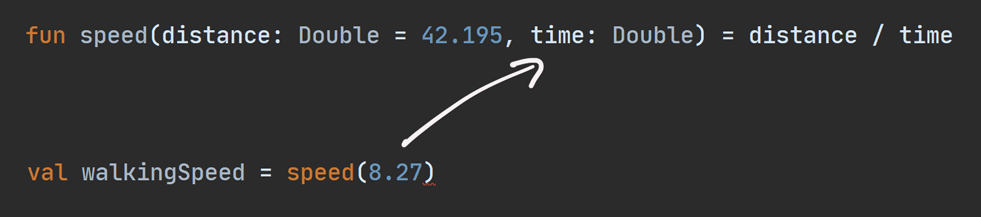But we actually assigned `8.27` to the `distance` parameter, because `8.27` is the first argument, and `distance` is the first parameter: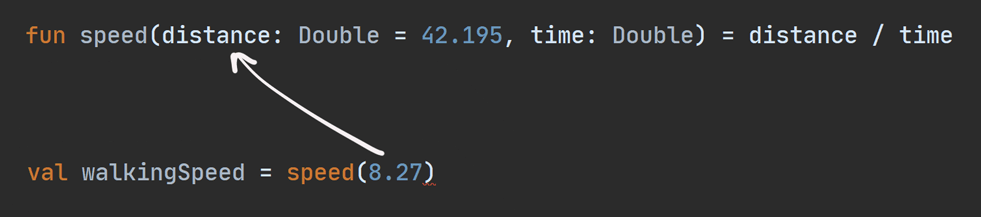To tell Kotlin that we’re sending the time instead, we simply use named arguments, like this:

``````val walkingSpeed = speed(time = 8.27)
val bikingSpeed = speed(time = 2.85)
val drivingSpeed = speed(time = 0.37)
val flyingSpeed = speed(0.12, 0.01)``````

Now, the first parameter, `distance`, will default to `42.195` when we call `speed()` for walking, biking, and driving.

## Expression Bodies and Block Bodies

So far we’ve been writing functions that just evaluate an expression.

• Our `circumference()` function just evaluates `2 * pi * radius`
• Our `speed()` function just evaluates `distance / time`

Let’s look at our code for `circumference()` again:

``````val pi = 3.14

When we write a function this way, where the body is just a single expression, we say that the function has an expression body.

Kotlin also gives us a second way that we can write functions. Let’s rewrite the `circumference()` function using this second way:

``````val pi = 3.14

return 2 * pi * radius
}``````

When we write a function like this, we call it a function with a block body.

The way you write a function with a block body is a little more complex than the way we’ve written expression body functions so far, so let’s take a closer look at the new pieces:

First, notice the `: Double` after the parentheses. We were able to use type inference for expression bodies, but when we use a block body, we must specify the return type explicitly.

Next, notice the opening and closing braces: `{` and `}`. Everything between those two braces is referred to as a code block (which is why we call this a function with a block body!)

Finally, notice the word `return` inside that code block. The word `return` is a keyword that tells Kotlin that the expression that follows it is what the function should return. As with expression body functions, the value that the function returns must match the type that we said the function returns!Functions with a block body take more typing than functions with an expression body, so why would we ever want to use them?

• They let you write more than one line of code in the function.
• They let you write statements inside them, not just expressions.

For example, so far we have defined `pi` outside of our function. But it’d be great to define it inside the function instead:

``````fun circumference(radius: Double): Double {
val pi = 3.14
return 2 * pi * radius
}``````

By moving `pi` to the inside of the function like this, it will only be accessible from inside the function. In other words, if you try to use it outside of the function, you’ll get an error.

``````fun circumference(radius: Double): Double {
val pi = 3.14
return 2 * pi * radius
}

val tau = 2 * pi``````
Error

Throughout the rest of this book, we’ll see many examples of both functions with expression bodies and functions with block bodies.

## Functions without a Result

There are times when you might want a function that doesn’t return a result. For example, let’s say we have a variable that holds a number that increases over time, named `counter`. We’ll create a function named `increment()`. Every time we call that function, it’ll increase `counter` by one, by writing the statement `counter = counter + 1`.

Functions with expression bodies can only contain a single expression. We cannot use a statement in an expression body. For example, we can’t do this:

``````var counter = 0

fun increment() = counter = counter + 1``````
Error

Instead of using an expression body, it’s better to use a block body for this function instead:

``````var counter = 0

fun increment() {
counter = counter + 1
}``````

You probably noticed that we didn’t specify a return type on this function.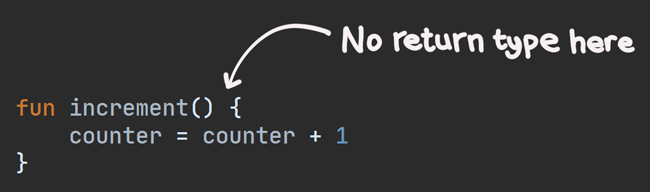You might be surprised to learn that, even though we didn’t specify a return type, and even though this function contains no expression, this function still returns a value!

That’s right! When you omit a return type for a function that has a block body, it automatically returns a special Kotlin type called `Unit`.

`Unit` - it’s not a number or string, and you can’t really do much with it. But it ends up being helpful in some cases that we’ll explore when we get to generics in a future chapter.

But for now, let’s wrap up the current chapter!

## Summary

In this chapter, we learned:

Next up in chapter 3, we’ll learn all about conditionals in Kotlin, so that we can get our code to do different things in different situations. See you then!

Thanks to Louis CAD, James Lorenzen, Matt McKenna, and Charles Muchene for reviewing this chapter.